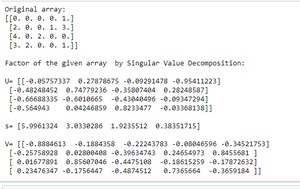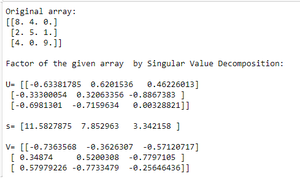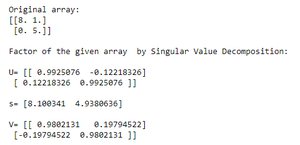# Compute the factor of a given array by Singular Value Decomposition using NumPy

• Last Updated : 29 Aug, 2020

Singular Value Decomposition means when arr is a 2D array, it is factorized as u and vh, where u and vh are 2D unitary arrays and s is a 1D array of a’s singular values. numpy.linalg.svd() function is used to compute the factor of an array by Singular Value Decomposition.

Syntax : numpy.linalg.svd(a, full_matrices=True, compute_uv=True, hermitian=False)

Parameters :

• a (…, M, N) array : A real or complex array with a.ndim >= 2.
• full_matrices(bool, optional) : If True (default), u and vh have the shapes (…, M, M) and (…, N, N), respectively. Otherwise, the shapes are (…, M, K) and (…, K, N), respectively, where K = min(M, N).
• compute_uv(bool, optional) : Whether or not to compute u and vh in addition to s. Its default value is True.
• hermitian(bool, optional) : If True, a is assumed to be Hermitian (symmetric if real-valued), enabling a more efficient method for finding singular values. Its default value is False.

Below are some examples on how to use the function :

Example 1 :

## Python3

 `# Import numpy library``import` `numpy as np`` ` `# Create a numpy array``arr ``=` `np.array([[``0``, ``0``, ``0``, ``0``, ``1``], [``2``, ``0``, ``0``, ``1``, ``3``],``                ``[``4``, ``0``, ``2``, ``0``, ``0``], [``3``, ``2``, ``0``, ``0``, ``1``]],``               ``dtype``=``np.float32)`` ` `print``(``"Original array:"``)``print``(arr)`` ` `# Compute the factor by Singular Value ``# Decomposition``U, s, V ``=` `np.linalg.svd(arr, full_matrices``=``False``)`` ` `# Print the result``print``(``"\nFactor of the given array  by Singular Value Decomposition:"``)``print``(``"\nU="``, U, ``"\n\ns="``, s, ``"\n\nV="``, V)`

Output :Example 2 :

## Python3

 `# Import numpy library``import` `numpy as np`` ` `# Create a numpy array``arr ``=` `np.array([[``8``, ``4``, ``0``], [``2``, ``5``, ``1``], ``                ``[``4``, ``0``, ``9``]], dtype``=``np.float32)`` ` `print``(``"Original array:"``)``print``(arr)`` ` `# Compute the factor ``U, s, V ``=` `np.linalg.svd(arr, full_matrices``=``False``)`` ` `# Print the result``print``(``"\nFactor of the given array  by Singular Value Decomposition:"``)``print``(``"\nU="``, U, ``"\n\ns="``, s, ``"\n\nV="``, V)`

Output :Example 3 :

## Python3

 `# Import numpy library``import` `numpy as np`` ` `# Create a numpy array``arr ``=` `np.array([[``8``, ``1``], [``0``, ``5``]], dtype``=``np.float32)``print``(``"Original array:"``)``print``(arr)`` ` `# Compute the factor ``U, s, V ``=` `np.linalg.svd(arr, full_matrices``=``False``)`` ` `# Print the result``print``(``"\nFactor of the given array  by Singular Value Decomposition:"``)``print``(``"\nU="``, U, ``"\n\ns="``, s, ``"\n\nV="``, V)`

Output :My Personal Notes arrow_drop_up